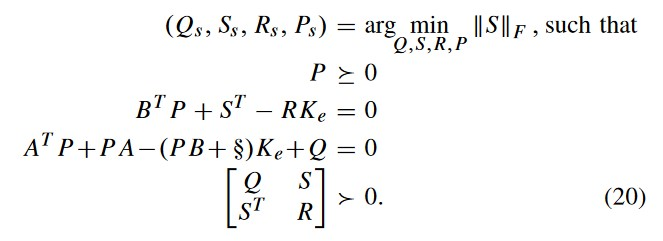# Implementation of LMI problem in cvx%% Matrix A,B,C
A=[100 0 -1;0 0.1 50;0.333 10 0];
B=[-1 0 10;1 1 0;0.1 -20 4];
C=eye(3);
K=[-3.47 20.2 49.3;3.7 0.0519 0.714;18.7 2.21 4.83];
cvx_begin sdp
variable S
variable P(3,3) symmetric
variable Q(3,3) symmetric
variable R(3,3) symmetric
variable d(3,3) symmetric
minimise(norm(S))
P >= 0;
B’P+S’-RK0== 0;
A’P + PA-(P*B+d)*K+Q==0;
Q > 0 ;
S > 0;
S’> 0;
R >= 0 ;
cvx_end
I need to implement above LMI using cvx, the codes I used are shown above but I am not getting correct final value.

You haven’t implemented the model as shown.

You are missing the LMI: `[Q S;S'R] > 0` But actually, CVX treats `>` as `>=`. So if you need strict inequality (i.e., positive definiteness), then you’ll need something like
`[Q S;S'R] - small_positive_number*eye(6) >= 0` , where `small_positive_number` is perhaps about `1e-5`.

And you will need to declare `variable S(3,3)`, not `variable S`.
And remove `S > 0`.

And you can omit `Q > 0` and `R >= 0`

Also, you need `minimise(norm(S,'fro'))` rather than the 2-norm which is used by default with `norm`.

I’ll let you check to see whether I missed anything.

%% Matrix A,B,C
A=[100 0 -1;0 0.1 50;0.333 10 0];
B=[-1 0 10;1 1 0;0.1 -20 4];
C=eye(3);
K0=[-3.47 20.2 49.3;3.7 0.0519 0.714;18.7 2.21 4.83];
cvx_begin sdp
variable S(3,3) symmetric
variable P(3,3) symmetric
variable Q(3,3) symmetric
variable R(3,3) symmetric
variable d(3,3) symmetric
minimise(norm(S,‘fro’))
P >= 0;
B’P+S’-RK0== 0;
A’P + PA-(P*B+d)K0+Q==0;
[Q S;S’ R] - 1e-5
eye(6) >= 0;

For this result is
Status: Inaccurate/Solved
Optimal value (cvx_optval): +9.02486e-05
Q =

``````1.9880    1.6727    2.4909
1.6727    4.0435    5.6830
2.4909    5.6830    9.2900
``````

R

R =

``````0.0110   -0.2243    0.0116
``````

-0.2243 6.5938 -1.3415
0.0116 -1.3415 0.6180

I am trying to implement one example in the paper “Solutions to the Inverse LQR Problem With Application to Biological Systems Analysis”. In this initial value for Q and R are estimated using the above LMI.
Answer given in the paper is
Q=
1.0e+03 *

``````0.0714    0.0964    0.0628
0.0964    0.3020    0.0086
0.0628    0.0086    1.5500
``````

R=
R =

``````1.3300   -1.4900   -3.1900
``````

-1.4900 387.0000 -77.0000
-3.1900 -77.0000 53.3000

I don’t believe `S` should be declared to be symmetric.